# HICROP in R

HICROP input variables include topographic, edaphic, and climatic variables to identify spatial niche suitability for selected crops.

The topographic and edaphic variables are: slope, soil depth, soil drainage, and soil pH.

First, make some basic functions

# Model funcitons

``````# Call package libraries
library(rgdal)
library(RColorBrewer)
library(raster)
library(dplyr)
library(tidyverse)
library(pbapply)

# # Local variables:
# # function to prompt user to input crop name
#   return(toString(n))
#   }
#
# # PROMPTS USER TO INPUT CROP NAME
# crop <-  readstring() #Input, String

#crop_db <-  read.csv("CROPS.csv", stringsAsFactors = F) #insert csv file path here

# # Use col name of variable to index crop_db and return value
# locate <- function(var) {
#   n <- eval(parse(text=(paste0("crop_db\$","P_OPTMIN","[na.omit(crop_db\$NAME == crop)]"))))
#   return(n)
# }

# General suitability function
suit4 <- function(x) { ifelse(x < ABSmin, 0, # Conditional statement for values less than absolute minimum
ifelse(x > ABSmax, 0, # Conditional statement for values greater than absolute maximum
ifelse(((x >= OPTmin) & (x <= OPTmax)),100, # Conditional statement for values greater than or equal absolute maximum AND less than or equal to optimal maximum (i.e. the ideal zone)
ifelse(((x <= ABSmax) & (x > OPTmax)),((1-(x-OPTmax)/(ABSmax-OPTmax))*100), # Conditional statement for values less than or equal absolute maximum AND greater than optimal maximum
ifelse(((x >= ABSmin) & (x < OPTmin)),(x-ABSmin)/(OPTmin-ABSmin)*100,NA ) )))) } # Conditional statement for values greater than or equal absolute minimum AND less than optimal maximum
``````

# import crop db

``````# write.csv(crop_db, "crop_database_07_28_2019.csv")
#
#  crop_dba <- crop_db
#
# crop_db <- read.csv("crop_database_07_28_2019.csv", stringsAsFactors = FALSE)
#
# crop_db\$X <- NULL
``````

# Select crop

``````# crop defining tool
crop_define <- function() {
n <- readline(prompt="Enter crop name: ")
m <- crop_db
ind <- grep(pattern = n,x = m\$species,ignore.case = TRUE)
ind <- ifelse(length(ind) == 0, grep(pattern = n,x = m\$Common_names,ignore.case = TRUE), ind)
if(length(ind) == 1) {
print(paste0("You have selected: ", m[ind,]\$species, " AKA ", strsplit(x = m[ind,67],", ")[]))
n <- ind
return(select(m[n,], crop_code,NAME, species, Common_names, W_OPT_min:C_MAX,Habit, Life.form, Life.span))
} else if(length(ind) > 1) {
print("There are multiple potential crops. Please reveiw the list")
print(m[ind,] %>% select(species, Common_names))
n <- readline(prompt="There are multiple potential crops. Please reveiw the list and enter the desired CROP CODE: ")
print(paste0("You have selected: ", m[n,]\$species, " AKA ", strsplit(x = m[n,67],", ")[]))
return(select(m[n,], crop_code,NAME, species, Common_names, W_OPT_min:C_MAX,Habit, Life.form, Life.span))
} else {
if(length(ind) == 0) print(paste0(n," is not in the crop database."))
}
}

crop_vars <- crop_define()
``````
```##  "You have selected: Artocarpus altilis AKA breadfruit"
```
``````crop <- crop_vars\$NAME
#View(crop_vars)

#rm(crop_vars)
``````

# Start timer

``````# Start the clock!
ptm <- proc.time()
``````

# Define crop parameters & output file

``````setwd("/Users/hh/Documents/GitHub/HICROP/Outputs/")
``````
```## Error in setwd("/Users/hh/Documents/GitHub/HICROP/Outputs/"): cannot change working directory
```
``````make.dir <- function(fp) {
if(!file.exists(fp)) {  # If the folder does not exist, create a new one
make.dir(dirname(fp))
dir.create(fp)
} else {   # If it existed, delete and replace with a new one
dir.create(fp)
}
}

make.dir(paste0(crop,sep="_",Sys.Date()))
``````
```## Warning in dir.create(fp): '.' already exists
```
``````result_path <- paste0("Outputs/",crop,sep="_",Sys.Date())
``````

# Import Raster Files

``````setwd("/Users/hh/Documents/GitHub/HICROP")
``````

# Save crop parameters to output file

``````# save crop parameters to file
write.csv(crop_vars, paste0(paste0(result_path, "/params.csv")))
``````
```## Warning in file(file, ifelse(append, "a", "w")): cannot open file 'Outputs/
## breadfruit_2019-07-29/params.csv': No such file or directory
```
```## Error in file(file, ifelse(append, "a", "w")): cannot open the connection
```

# Ph Suitability

``````PH <- raster("inputs/toMac/ph.tif")
``````
```## Error in .local(.Object, ...) :
```
```## Error in .rasterObjectFromFile(x, band = band, objecttype = "RasterLayer", : Cannot create a RasterLayer object from this file. (file does not exist)
```
``````# get variables
OPTmin <- crop_db\$P_OPTMIN[crop_db\$crop_code == crop_vars\$crop_code]
OPTmax <- crop_db\$P_OPTMAX[crop_db\$crop_code == crop_vars\$crop_code]
ABSmin <- crop_db\$P_ABSMIN[crop_db\$crop_code == crop_vars\$crop_code]
ABSmax <- crop_db\$P_ABSMAX[crop_db\$crop_code == crop_vars\$crop_code]

print(paste("Optimal soil pH range for", crop,"is:", OPTmin,"-",OPTmax))
``````
```##  "Optimal soil pH range for breadfruit is: 5.5 - 6.5"
```
``````print(paste("Absolute soil pH range for", crop,"is:", ABSmin,"-",ABSmax))
``````
```##  "Absolute soil pH range for breadfruit is: 4.3 - 8.7"
```
``````# calculate suitability
P_suit <- calc(PH, suit4)
``````
```## Error in calc(PH, suit4): object 'PH' not found
```
``````# plot output
plot(P_suit, main= paste("Ph Suitability for ",crop), breaks = c(0,25,50,75,100), col = rainbow(5),
sub=paste("Optimal pH range:", OPTmin,"-",OPTmax, "\n Absolute pH range:", ABSmin,"-",ABSmax))
``````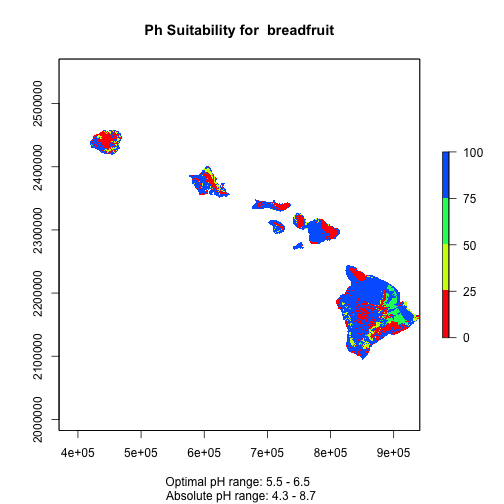``````# cleanup & save
writeRaster(P_suit, filename = paste0("Outputs/",crop,sep="_",Sys.Date(),"/ph_suit.tif"))
``````
```## Error in .local(x, filename, ...): Attempting to write a file to a path that does not exist:
```
``````rm(PH)
``````
```## Warning in rm(PH): object 'PH' not found
```

A primary difference between the two is that the python script performs multiple condtional operations (i.e., rastcon1 through rastcon5) and output operations (i.e., true3 and true4) for each input value, before determining which conditional and output apply (see rast_suit, line 138). The R code reduces the total number of calculations by only determining outputs once a conditional is met (see soilsuit, lines 202-206). This truncating approach is taken for all scripts below.

Other than that the only differences are the that the python scripts pull in parameter data from a csv whereas currently only dummy data was selected and hardcorded in (e.g., ph_ABSmax etc).

# Depth Suitability

``````DEPTH <- raster("inputs/toMac/depths.tif")
``````
```## Error in .local(.Object, ...) :
```
```## Error in .rasterObjectFromFile(x, band = band, objecttype = "RasterLayer", : Cannot create a RasterLayer object from this file. (file does not exist)
```
``````#freq(DEPTH)

# Get variables
OPTmin <- crop_db\$D_OPT[crop_db\$crop_code == crop_vars\$crop_code]
ABSmin <- crop_db\$D_ABS[crop_db\$crop_code == crop_vars\$crop_code]

print(paste("Optimal minimum soil depth for", crop,"is:", OPTmin, "cm"))
``````
```##  "Optimal minimum soil depth for breadfruit is: 150 cm"
```
``````print(paste("Absolute minimum soil depth for", crop,"is:", ABSmin, "cm"))
``````
```##  "Absolute minimum soil depth for breadfruit is: 20 cm"
```
``````# # define custom function
# suit2min <- function(x) { ifelse(x < ABSmin, 0, # Conditional statement for values less than absolute minimum
#                                  ifelse(x >= OPTmin, 100, # Conditional for values greater than or equal to optimal minimum
#                                         ifelse(((x >= ABSmin) & (x < OPTmin)), ((x-ABSmin)/(OPTmin-ABSmin)*100), NA)))}
#
# # run calculation
# D_suit <- calc(DEPTH, suit2min)

# use pre-run suitability stack
D_suit <- depth_stack[[which(apply(dep, 1, function(r) any(r %in% OPTmin & r %in% ABSmin)))]]

# plot output
plot(D_suit, main= paste("Depth Suitability for ",crop), breaks = c(0,25,50,75,100), col = rainbow(5),
sub = paste('Optimal minimum depth is ', OPTmin, 'cm, \n Absolute minimum depths is', ABSmin, 'cm.'))
``````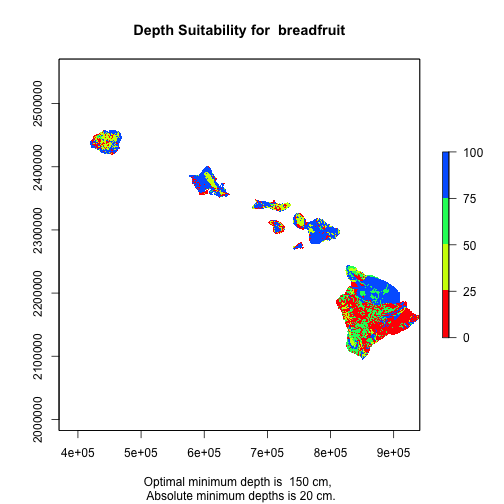``````# save and cleanup
writeRaster(D_suit, filename = paste0("Outputs/",crop,sep="_",Sys.Date(),"/depth_suit.tif"))
``````
```## Error in .local(x, filename, ...): Attempting to write a file to a path that does not exist:
```
``````#rm(DEPTH)
``````

# Drainage Suitability

``````#DRAIN <- raster("inputs/toMac/drain.tif")
#freq(DRAIN)

# get variables
OPTmin <- crop_db\$W_OPT_min[crop_db\$crop_code == crop_vars\$crop_code]
OPTmax <- crop_db\$W_OPT_max[crop_db\$crop_code == crop_vars\$crop_code]
ABSmin <- crop_db\$W_ABS_min[crop_db\$crop_code == crop_vars\$crop_code]
ABSmax <- crop_db\$W_ABS_max[crop_db\$crop_code == crop_vars\$crop_code]

print(paste("Optimal soil drainage code range for", crop,"is:", OPTmin,"-",OPTmax))
``````
```##  "Optimal soil drainage code range for breadfruit is: 6 - 6"
```
``````print(paste("Absolute soil drainage code range for", crop,"is:", ABSmin,"-",ABSmax))
``````
```##  "Absolute soil drainage code range for breadfruit is: 6 - 6"
```
``````# calculate suitability
#W_suit <- calc(DRAIN, suit4)

# use pre-run suitability stack
W_suit <- drain_stack[[which(apply(dra, 1, function(r) any(r %in% OPTmin & r %in% OPTmax & r %in% ABSmin & r %in% ABSmax)))]]

plot(W_suit, main= paste("Drainage Suitability for ",crop), breaks = c(0,25,50,75,100), col = rainbow(5),
sub=paste("Optimal drainage range:", OPTmin,"-",OPTmax, "\n Absolute drainage range:", ABSmin,"-",ABSmax))
``````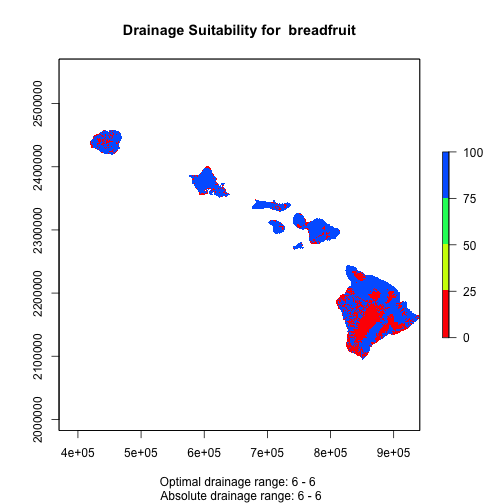``````# save and cleanup
writeRaster(W_suit, filename = paste0("Outputs/",crop,sep="_",Sys.Date(),"/drainage_suit.tif"))
``````
```## Error in .local(x, filename, ...): Attempting to write a file to a path that does not exist:
```
``````#rm(DRAIN)
``````

# Slope Suitability

``````# SLOPE <- raster("inputs/toMac/slope.tif")

# Determine slope input variables
OPTmax <- crop_db\$S_OPTMAX[crop_db\$crop_code == crop_vars\$crop_code]
ABSmax <- crop_db\$S_ABSMAX[crop_db\$crop_code == crop_vars\$crop_code]

# Reivew texts
print(paste("Optimal maximum slope for", crop,"is:", OPTmax, "%"))
``````
```##  "Optimal maximum slope for breadfruit is: 28 %"
```
``````print(paste("Absolute maximum soil slope for", crop,"is:", ABSmax, "%"))
``````
```##  "Absolute maximum soil slope for breadfruit is: 38 %"
```
``````#
# # Use fuzzy calculation to determine range of suitability for
# suit2max <- function(x) { ifelse(x > ABSmax, 0, # Conditional statement for values greater than absolute maximum slope
#                                   ifelse(x <= OPTmax, 100, # Conditional statement for values less than or equal to optimal maximum slope
#                                          ifelse(((x <= ABSmax) & (x > OPTmax)),((1-(x - OPTmax)/(ABSmax-OPTmax))*100), NA)))} # Conditional statement for values greater than or equal absolute minimun AND less than optimal minimum
#
# # calculate suitability
# S_suit <- calc(SLOPE, suit2max)

plot(S_suit, main= paste("Slope Suitability for ",crop), breaks = c(0,25,50,75,100), col = rainbow(5),
sub = paste('Optimal maximum slope is ', OPTmax, '%, \n Absolute maximum slope is', ABSmax, '%.'))
``````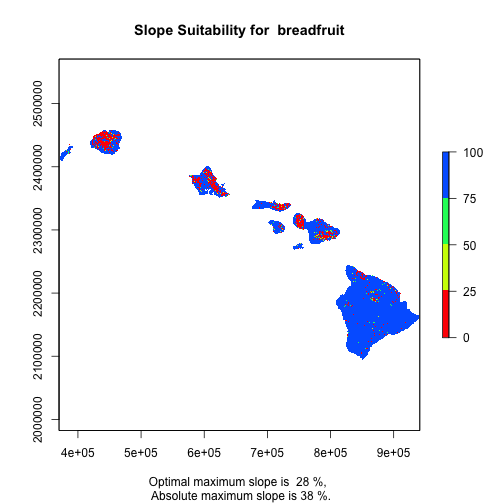``````# save and cleanup
writeRaster(S_suit, filename = paste0("Outputs/",crop,sep="_",Sys.Date(),"/slope_suit.tif"))
``````
```## Error in .local(x, filename, ...): Attempting to write a file to a path that does not exist:
```
``````#rm(SLOPE)
``````

# Calculate Temperature

``````# Get temp rasters
# get file list
# store as raster stack

Env_Tmax <- stack(sapply(Sys.glob("inputs/toMac_climate/tmax/*.tif"), raster))
``````
```## Error in x[]: subscript out of bounds
```
``````Env_Tmin <- stack(sapply(Sys.glob("inputs/toMac_climate/tmin/*.tif"), raster))
``````
```## Error in x[]: subscript out of bounds
```
``````# Get float values for temperature variables from ecocrop db
ABSmax <- crop_db\$T_ABSMAX[crop_db\$crop_code == crop_vars\$crop_code]
ABSmin <- crop_db\$T_ABSMIN[crop_db\$crop_code == crop_vars\$crop_code]
OPTmax <- crop_db\$T_OPTMAX[crop_db\$crop_code == crop_vars\$crop_code]
OPTmin <- crop_db\$T_OPTMIN[crop_db\$crop_code == crop_vars\$crop_code]

print(paste("Optimal Temperature range for", crop,"is:", OPTmin,"-",OPTmax, "°C"))
``````
```##  "Optimal Temperature range for breadfruit is: 21 - 33 °C"
```
``````print(paste("Absolute Temperature range for", crop,"is:", ABSmin,"-",ABSmax, "°C"))
``````
```##  "Absolute Temperature range for breadfruit is: 16 - 40 °C"
```
``````## Suitability Evaluation
Tmax <- calc(Env_Tmax, suit4)
``````
```## Error in .local(.Object, ...):
```
``````Tmin <- calc(Env_Tmin, suit4)
``````
```## Error in .local(.Object, ...):
```
``````# make a raster stack with minimum value from min and max suitability layers by month

f <- function(x1, x2)  ifelse(x1 < x2, x1, x2)
T_suit <- overlay(Tmax, Tmin, fun=f, forcefun=TRUE)

#plot(T_suit, main= paste0("Temperature Suitability for ", crop), breaks = c(0,25,50,75,100), col = rainbow(5))

library(rasterVis)

levelplot(T_suit, main= paste0("Temperature Suitability for ", crop),
names.attr=month.name[1:12],
sub=paste("Optimal Temperature range:", OPTmin,"-",OPTmax, "°C \n Absolute Temperature range:", ABSmin,"-",ABSmax, "°C"),
at = seq(0, 100, by = 20),
col.regions = rainbow(5))
``````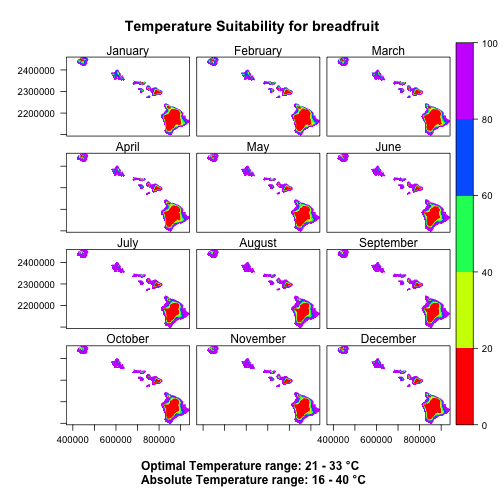# Rainfall suitability analysis

``````# get list of Tif filenames and make raster stack
# NEED TO FIGURE OUT WHY THIS BROKE
# Env_rain <- sapply(Sys.glob("inputs/rainfall/*.tif"), raster) %>% stack()
Env_rain <- stack(sapply(Sys.glob("inputs/toMac_climate/rain/*.tif"), raster))
``````
```## Error in x[]: subscript out of bounds
```
``````# get variables
ABSmax <- round(crop_db\$R_ABSMAX[crop_db\$crop_code == crop_vars\$crop_code]/12,2)
ABSmin <- round(crop_db\$R_ABSMIN[crop_db\$crop_code == crop_vars\$crop_code]/12,2)
OPTmax <- round(crop_db\$R_OPTMAX[crop_db\$crop_code == crop_vars\$crop_code]/12,2)
OPTmin <- round(crop_db\$R_OPTMIN[crop_db\$crop_code == crop_vars\$crop_code]/12,2)

# Get float values for precipitation variables from ecocrop csv file:
print(paste("Optimal Precipitation range for", crop,"is:", OPTmin,"-",OPTmax, "mm"))
``````
```##  "Optimal Precipitation range for breadfruit is: 125 - 250 mm"
```
``````print(paste("Absolute Precipitation range for", crop,"is:", ABSmin,"-",ABSmax, "mm"))
``````
```##  "Absolute Precipitation range for breadfruit is: 83.33 - 291.67 mm"
```
``````# calculate suitability
R_suit <- calc(Env_rain, suit4)
``````
```## Error in .local(.Object, ...):
```
``````# plot outputs
levelplot(R_suit, main= paste0("Precipitation Suitability for ", crop),
names.attr=month.name[1:12],
sub=paste("Optimal Precipitation range:", round(OPTmin),"-",round(OPTmax), "mm \n Absolute Precipitation range:", round(ABSmin),"-",round(ABSmax), "mm"),
at = seq(0, 100, by = 20),
col.regions = rainbow(5))
``````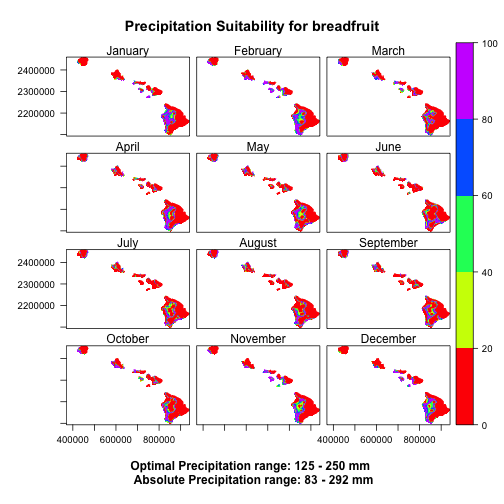# Seasonal Calculations

``````# Get the max days of season,
# divide and round up to produce integer for season length in months

#Cmin <- as.integer(ceiling(locate("C_MIN")/30.42))
Cmax <- as.integer(ceiling(crop_db\$C_MAX[crop_db\$crop_code == crop_vars\$crop_code]/30.42)) # 365/12=30.42

print(paste("Maximum growing season length for", crop,"is:", Cmax, "months"))
``````
```##  "Maximum growing season length for breadfruit is: 12 months"
```
``````#MAKE ONE GIANT SEASON ASSESSMENT FUNCTION

# Calculate Average Suitability and Difference across months in a season
season_avg <- function(var){
# make a double stack of monthly input variable (R or T)
# this will allow for calculating across all season lenghts (e.g., 12 month season starting in December..)
months24 <- stack(var, var)

# Make a 2-row matrix of all growing season possibiliites, based on maximum season length
# index season by col
seasons <- sapply(1:12, function(n) c(n, n+Cmax-1))
# make a list of stack
z <- sapply(1:12, function(i) months24[[seasons[1,i]:seasons[2,i]]])

# calculate seasonal means
agg.fun <- function(x,...) { ## you should add ... for na.rm,and other extra parameters
# if Cmax >= 12, run only the first season stack mean, else run mean for each season
if (Cmax >= 12){return(calc(x[], mean)) # if crop cycle lengths is 12 or more months, take the mean of year suitability
print("Calculating mean season")
}else{ # else, make mean of all seasons
print("Calculating mean seasons")
return(stack(pbsapply(1:12, function(i) calc(z[i][], mean))))  # REPLACE wih sapply(1:12,... and remove stack?
}
}

seasons_mean <- agg.fun(z)
return(seasons_mean)
}

#Check if RAINFALL / TEMP is annual or monthly
Rcat <- if (dim(R_suit) == 12){
print("Evaluating RAINFALL suitability by season")
season_avg(R_suit)
}
``````
```##  "Evaluating RAINFALL suitability by season"
```
``````Tcat <- if (dim(T_suit) == 12){
print("Evaluating TEMPERATURE suitability by season")
season_avg(T_suit)
}
``````
```##  "Evaluating TEMPERATURE suitability by season"
```
``````#Create Rain by Temp suitability
rainxtemp <- function(x,y){
rxt_stack <- stack() # make and empty raster stack
rast_stack <- stack(x,y) # stack two input raster stacks (Rcat & Tcat) as one
if(dim(rast_stack) == 24){ # if season is less than 12 months
print("Calculating Rain by Temp suitability across multiple seasons")
for(i in 1:12){ # for each season mean pairing
print(paste0("Calculating Rain by Temp suitability for season ", i))
rxt <- (rast_stack[[i]] * rast_stack[[i + 12]]) / 100 # factor season mean pairs (Rcat*Tcat)
rxt_stack <- stack(rxt_stack, rxt)} # store result in new stack
}
else { #if Cmax >= 12, all seasons will be the same (i.e. there is no need to loop through additional seasons)
print("Calculating Rain by Temp for complete year")
fun <- function(z) { ((z[] * z[]) / 100)}
rxt_stack <- calc(rast_stack, fun) # store result in new stack
}
return(rxt_stack) # return new stack
}

#if TEMP & RAIN is monthly, then run the rainxtemp function defined above
RxxT <- if((dim(R_suit) == 12) & (dim(T_suit) == 12)) rainxtemp(Rcat, Tcat)
``````
```##  "Calculating Rain by Temp for complete year"
```
``````# plot and save if Cmax >=12 aka 12+ month season
if (dim(RxxT)==1) plot(RxxT)
``````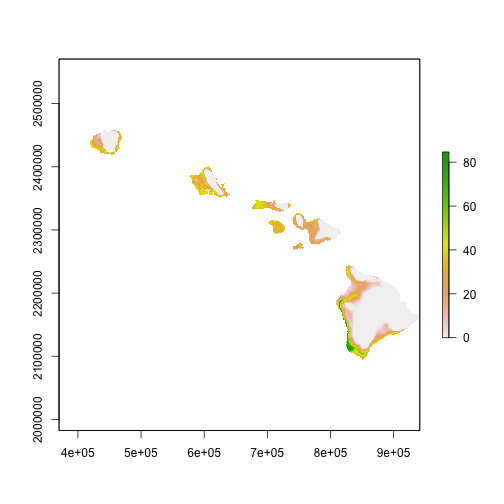``````if (dim(RxxT)==1) writeRaster(RxxT, paste0(result_path, "/RxT_suit.tif"))
``````
```## Error in .local(x, filename, ...): Attempting to write a file to a path that does not exist:
```

# Final Suitability Overlay & 0ptimal Planting Month

``````if (dim(T_suit) == 12) print("Temp is monthly (seasonal")
``````
```##  "Temp is monthly (seasonal"
```
``````if (dim(T_suit) == 1) print("Temp is annual")
if (dim(R_suit) == 12) print("Precip is monthly (seasonal")
``````
```##  "Precip is monthly (seasonal"
```
``````if (dim(R_suit) == 1) print("Precip is annual")

#if either rainfall or temperature is seasonal {ANNUAL?}, determine optimal season
if (any((dim(R_suit) == 12),(dim(T_suit) == 12))) {
#if both rainfall and temperature are monthly
# use RxT raster catalog created by "Seasons" script
if ((dim(R_suit) == 12) & (dim(T_suit) == 12)) {
seasonal <- RxxT
} else if((dim(R_suit) == 1) & (dim(T_suit) == 12)) {
seasonal <- T_suit
} else if((dim(R_suit) == 12) & (dim(T_suit) == 1)) {
seasonal <- R_suit
}

#Determine Optimal Season
# make function to find index of max cell in stack
which.max.na <- function(x, ...) ifelse(length(x) == sum(is.na(x)), 0, which.max(x))
# run
opt_season <- calc(seasonal, which.max.na)
#set all pixels that are not contained in month_nums to NA
opt_season[!(opt_season %in% c(1:12))] <- NA

#Return MAXIMUM Score (from optimal season)
seasuit <- max(seasonal)
print ("Maximum Seasonal (Temp and/or Rain) Suitability Score Raster: seasuit")

} else print("All criteria are annual")
``````
```## Warning in max(new("RasterLayer", file = new(".RasterFile", name = "/
## private/var/folders/xw/8jnc9qm53jz_gn09wx6tc3yr0000gn/T/RtmpWAr4ia/raster/
## r_tmp_2019-07-29_113539_42736_45557.grd", : Nothing to summarize if you
## provide a single RasterLayer; see cellStats
```
```##  "Maximum Seasonal (Temp and/or Rain) Suitability Score Raster: seasuit"
```
``````# # RESAMPLING TO BE REMOVED
# Psuit <- resample(P_suit,seasuit,method='ngb')
# Dsuit <- resample(D_suit,seasuit,method='ngb')
# Ssuit <- resample(S_suit,seasuit,method='ngb')
# Wsuit <- resample(W_suit,seasuit,method='ngb')
#
#
# #Overall MAXIMUM Score (all criteria included, across all available seasons)
# if ((dim(R_suit) == 12) & (dim(T_suit) == 12)) {
#   all_criteria <- stack(Dsuit, Psuit, Ssuit, Wsuit, seasuit)
# } else if((dim(R_suit) == 1) & (dim(T_suit) == 12)) {
#   all_criteria <- stack(Dsuit, Psuit, Ssuit, Wsuit, seasuit, annual_rainfall)
# } else if((dim(R_suit) == 12) & (dim(T_suit) == 1)) {
#   all_criteria <- stack(Dsuit, Psuit, Ssuit, Wsuit, seasuit, annual_temp)
# } else if ((dim(R_suit) == 1) & (dim(T_suit) == 1)) {
#   all_criteria <- stack(Dsuit, Psuit, Ssuit, Wsuit, annual_rainfall, annual_temp)
# } else print("error in at least one raster catalog, catalog should contain 1 or 12 rasters")
#

#Overall MAXIMUM Score (all criteria included, across all available seasons)
if ((dim(R_suit) == 12) & (dim(T_suit) == 12)) {
all_criteria <- stack(D_suit, P_suit, S_suit, W_suit, seasuit)
} else if((dim(R_suit) == 1) & (dim(T_suit) == 12)) {
all_criteria <- stack(D_suit, P_suit, S_suit, W_suit, seasuit, annual_rainfall)
} else if((dim(R_suit) == 12) & (dim(T_suit) == 1)) {
all_criteria <- stack(D_suit, P_suit, S_suit, W_suit, seasuit, annual_temp)
} else if ((dim(R_suit) == 1) & (dim(T_suit) == 1)) {
all_criteria <- stack(D_suit, P_suit, S_suit, W_suit, annual_rainfall, annual_temp)
} else print("error in at least one raster catalog, catalog should contain 1 or 12 rasters")

suit <- min(all_criteria)

plot(suit, main= paste("Suitability for ",crop), breaks = c(0,25,50,75,100), col = rainbow(5))
``````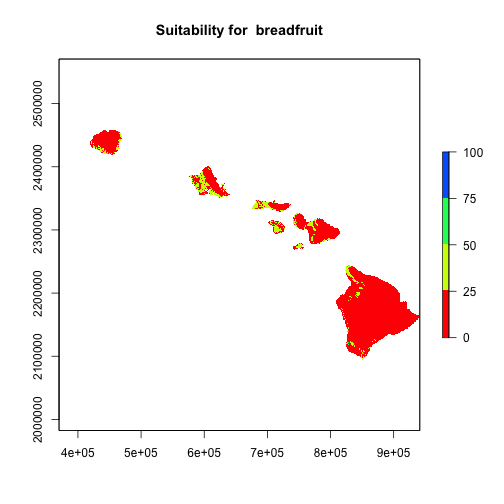``````# cleanup & save
writeRaster(suit, filename = paste0("Outputs/",crop,sep="_",Sys.Date(),"/all_suit.tif"))
``````
```## Error in .local(x, filename, ...): Attempting to write a file to a path that does not exist:
```
``````writeRaster(opt_season, filename = paste0("Outputs/",crop,sep="_",Sys.Date(),"/optimal_season.tif"))
``````
```## Error in .local(x, filename, ...): Attempting to write a file to a path that does not exist:
```

# Limiting Factors

``````print(paste("Maximum growing season length for", crop,"is:", Cmax, "months"))
``````
```##  "Maximum growing season length for breadfruit is: 12 months"
```
``````# ######
# #use a seasonal raster if it exists otherwise use a non-seasonal raster
# Rsuit_cat <- env.workspace +"/"+ crop[0:3] + "_Rsuit" + str(Cmax)
# if not arcpy.Exists(Rsuit_cat):
#   R_Rsuit = env.workspace +"/"+ crop[0:3] + "_Rsuit/Raster.OBJECTID=1"
#
# Tsuit_cat = env.workspace +"/"+ crop[0:3] + "_Tsuit" + str(Cmax)
# if not arcpy.Exists(Tsuit_cat):
#   R_Tsuit = env.workspace +"/"+ crop[0:3] + "_Tsuit/Raster.OBJECTID=1"
# ################

# If season is >= 12 months uses mean of R_suit stack (Rcat), else uses mean of first season stack (Rcat[])
### CHECK IF THESE TWO LINES ARE NEEDED
#Rc <- ifelse (dim(Rcat) == 12, Rcat[], Rcat)
#Rc <- ifelse (dim(Rcat) == 1, Rcat, Rcat[])

if (dim(Rcat) == 12) {
Rc <- Rcat[]
} else {
Rc <-Rcat
}
Rc
``````
```## class      : RasterLayer
## dimensions : 3663, 5712, 20923056  (nrow, ncol, ncell)
## resolution : 100, 100  (x, y)
## extent     : 370100, 941300, 2093300, 2459600  (xmin, xmax, ymin, ymax)
## crs        : +proj=utm +zone=4 +datum=NAD83 +units=m +no_defs +ellps=GRS80 +towgs84=0,0,0
## source     : /private/var/folders/xw/8jnc9qm53jz_gn09wx6tc3yr0000gn/T/RtmpWAr4ia/raster/r_tmp_2019-07-29_113203_42736_43794.grd
## names      : layer
## values     : 0, 90.93108  (min, max)
```
``````if (dim(Tcat) == 12) {
Tc <- Tcat[]
} else {
Tc <-Tcat
}
Tc
``````
```## class      : RasterLayer
## dimensions : 3663, 5712, 20923056  (nrow, ncol, ncell)
## resolution : 100, 100  (x, y)
## extent     : 370100, 941300, 2093300, 2459600  (xmin, xmax, ymin, ymax)
## crs        : +proj=utm +zone=4 +datum=NAD83 +units=m +no_defs +ellps=GRS80 +towgs84=0,0,0
## source     : /private/var/folders/xw/8jnc9qm53jz_gn09wx6tc3yr0000gn/T/RtmpWAr4ia/raster/r_tmp_2019-07-29_113354_42736_32893.grd
## names      : layer
## values     : 0, 99.96182  (min, max)
```
``````ss <- stack(P_suit, D_suit, W_suit, S_suit, Tc, Rc) # make stack of layers
names(ss) <- c("Ph","Depth","Drainage","Slope","Temp","Rain") # rename layers for no reason
mns <- whiches.min(ss) # get lowest value(s) by layer

#freq(mns) %>% View()
#plot(mns)
#####

#unique(mns)

# access raster attribute table
mns <- ratify(mns) # make a rat
#mns
rat <- levels(mns)[]
#rat

# make new column and substitue layer index num (1:6) for layer name strings
rat\$limit <- gsub(1, "PH_", rat\$ID)
rat\$limit <- gsub(2, "DEPTH_", rat\$limit)
rat\$limit <- gsub(3, "DRAIN_", rat\$limit)
rat\$limit <- gsub(4, "SLOPE_", rat\$limit)
rat\$limit <- gsub(5, "TEMP_", rat\$limit)
rat\$limit <- gsub(6, "RAIN_", rat\$limit)
rat
``````
```##        ID                           limit
## 1       1                             PH_
## 2       2                          DEPTH_
## 3       3                          DRAIN_
## 4       4                          SLOPE_
## 5       5                           TEMP_
## 6       6                           RAIN_
## 7      12                       PH_DEPTH_
## 8      13                       PH_DRAIN_
## 9      14                       PH_SLOPE_
## 10     15                        PH_TEMP_
## 11     16                        PH_RAIN_
## 12     23                    DEPTH_DRAIN_
## 13     24                    DEPTH_SLOPE_
## 14     25                     DEPTH_TEMP_
## 15     26                     DEPTH_RAIN_
## 16     34                    DRAIN_SLOPE_
## 17     35                     DRAIN_TEMP_
## 18     36                     DRAIN_RAIN_
## 19     45                     SLOPE_TEMP_
## 20     46                     SLOPE_RAIN_
## 21     56                      TEMP_RAIN_
## 22    123                 PH_DEPTH_DRAIN_
## 23    124                 PH_DEPTH_SLOPE_
## 24    125                  PH_DEPTH_TEMP_
## 25    126                  PH_DEPTH_RAIN_
## 26    134                 PH_DRAIN_SLOPE_
## 27    135                  PH_DRAIN_TEMP_
## 28    136                  PH_DRAIN_RAIN_
## 29    145                  PH_SLOPE_TEMP_
## 30    146                  PH_SLOPE_RAIN_
## 31    156                   PH_TEMP_RAIN_
## 32    234              DEPTH_DRAIN_SLOPE_
## 33    235               DEPTH_DRAIN_TEMP_
## 34    236               DEPTH_DRAIN_RAIN_
## 35    245               DEPTH_SLOPE_TEMP_
## 36    246               DEPTH_SLOPE_RAIN_
## 37    256                DEPTH_TEMP_RAIN_
## 38    345               DRAIN_SLOPE_TEMP_
## 39    346               DRAIN_SLOPE_RAIN_
## 40    356                DRAIN_TEMP_RAIN_
## 41    456                SLOPE_TEMP_RAIN_
## 42   1234           PH_DEPTH_DRAIN_SLOPE_
## 43   1235            PH_DEPTH_DRAIN_TEMP_
## 44   1236            PH_DEPTH_DRAIN_RAIN_
## 45   1245            PH_DEPTH_SLOPE_TEMP_
## 46   1246            PH_DEPTH_SLOPE_RAIN_
## 47   1256             PH_DEPTH_TEMP_RAIN_
## 48   1345            PH_DRAIN_SLOPE_TEMP_
## 49   1346            PH_DRAIN_SLOPE_RAIN_
## 50   1356             PH_DRAIN_TEMP_RAIN_
## 51   1456             PH_SLOPE_TEMP_RAIN_
## 52   2345         DEPTH_DRAIN_SLOPE_TEMP_
## 53   2346         DEPTH_DRAIN_SLOPE_RAIN_
## 54   2356          DEPTH_DRAIN_TEMP_RAIN_
## 55   2456          DEPTH_SLOPE_TEMP_RAIN_
## 56   3456          DRAIN_SLOPE_TEMP_RAIN_
## 57  12345      PH_DEPTH_DRAIN_SLOPE_TEMP_
## 58  12346      PH_DEPTH_DRAIN_SLOPE_RAIN_
## 59  12356       PH_DEPTH_DRAIN_TEMP_RAIN_
## 60  13456       PH_DRAIN_SLOPE_TEMP_RAIN_
## 61  23456    DEPTH_DRAIN_SLOPE_TEMP_RAIN_
## 62 123456 PH_DEPTH_DRAIN_SLOPE_TEMP_RAIN_
```
``````levels(mns) <- rat # assign levels based on new rat

#freq(mns@data@attributes\$limit)

#mns

writeRaster(mns,paste0(result_path,"/limits.tif"))
``````
```## Error in .local(x, filename, ...): Attempting to write a file to a path that does not exist:
```

# Validation: Ommision Rate & RMSE

``````# Name:         RMSE
# Purpose:      Calculate root mean square error based on known crop locations

library(dplyr)
library(raster)
# make shapefile of
baseline <- shapefile("~/GIS/GIS Files/2015AgBaseline.shp/2015AgBaseline.shp")

bs_crops <- baseline@data\$CropCatego %>% unique()

# check for crop in location file, save as raster if present
if (crop %in% bs_crops) {
print(paste0(crop," has location data. RMSE & OR will be calculated."))
crop_loc <- baseline %>% subset(., CropCatego %in% crop) %>% #subset shapefile to crop data
rasterize(., seasuit) #rasterize to other layer parameters
crop_loc[crop_loc >= 1] <- 1 # set values to 1
suitscore <- suit
# Calculate Root Mean Square Error (RMSE) for everywhere
rmse <- ((suitscore/100)-1)**2
# trim data to only areas with crop location
# get sum of RMSE values
rmse_sum <- cellStats(x = rmse,stat = 'sum')
# count cells with crop
rmse_count <- zonal(rmse, crop_loc, 'count')
# calc RMSE value
rmse_value <- rmse_sum/rmse_count[1,2]
# report
print(paste0(crop," RMSE = ", round(rmse_value,5)))
# Calculate Omission Rate (OR)
#reclassify values below above 0 to 1
suitscore[suitscore>0]=1
suitscore <- mask(suitscore, crop_loc)  # trim to just location data
or_sum <- cellStats(x = suitscore,stat = 'sum') # get sum of viable cells with crop present
or_value =  1-(or_sum/rmse_count[1,2])
print(paste0(crop," Omission Rate= ", round(or_value,5)))  # report
# create a table
rmse_table <- data.frame("Crop" = crop, "Count" = rmse_count[1,2] , "SUM_RMSE" = rmse_sum, "RMSE" = rmse_value, "SUM_OR" = or_sum, "OMISSION" = or_value)
print(rmse_table)
#save outputs & cleanup
print("Saving RMSE & OR outputs")
writeRaster(rmse, filename = paste0("Outputs/",crop,sep="_",Sys.Date(),"/rmse.tif"))
write.csv(rmse_table, paste0("Outputs/",crop,sep="_",Sys.Date(),"/RMSE_OR.csv"))
rm(suitscore, rmse, rmse_count, rmse_value, or_sum, or_value)
}else  {print(paste0("No location data for ",crop, ". RMSE & Omission Rate calculated for HDOA 2015 Baseline Study crops only"))
}
``````
```##  "No location data for breadfruit. RMSE & Omission Rate calculated for HDOA 2015 Baseline Study crops only"
```
``````# PAU!
``````

# Stop the clock and Tell me its over

``````# Stop the clock
print(paste0(round((proc.time() - ptm)/60,0), " minute runtime."))
``````
```##  "9 minute runtime."
```
``````notify(paste0("R is done, after a ", round((proc.time() - ptm)/60,0), " minute runtime."), speakIt=TRUE)
``````
```## Warning in system(cmd): error in running command
```